Tamilnadu State Board New Syllabus Samacheer Kalvi 11th Maths Guide Pdf Chapter 12 Introduction to Probability Theory Ex 12.4 Text Book Back Questions and Answers, Notes.

## Tamilnadu Samacheer Kalvi 11th Maths Solutions Chapter 12 Introduction to Probability Theory Ex 12.4

Question 1.
A factory has two Machines – I and II. Machines – I produce 60 % of items and Machine – II produces 40 % of the items of the total output. Further 2 % of the items produced by Machine – I are defective whereas 4 % produced by Machine -II are defective. If an item is drawn at random what is the probability that it is defective?
Let A1 be the event that items are produced by machine – I, A2 be the event that items are produced by machine – II.
Let B be the event of drawing a defective item. We have to find the total probability of event B. That is P(B) clearly A1 and A2 are mutually exclusive and exhaustive events.
∴ P(B) = P(A1) . P(B/A1) + P(A2) . P(B/A2)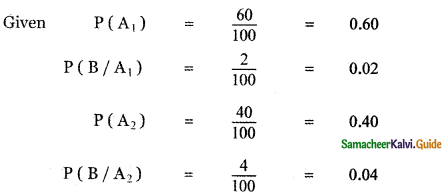P(B) = P(A1) . P(B/A1) + P(A2) . P(B/A2)
= 0.60 × 0.02 + 0.40 × 0.04
= 0.012 + 0.016
= 0.028Question 2.
There are two identical urns containing respectively 6 black and 4 red balls, 2 black, and 2 red balls. An urn is chosen at random and a ball is drawn from it.
(i) Find the probability that the ball is black
(ii) if the ball is black, what is the probability that it is from the first urn?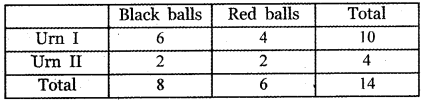(i) Let A1 be the event of selecting Urn – I and A2 be the event of selecting Urn – II.
Let B be the event of selecting one black ball.
We have to find the total probability of event B. That is P(B).
Clearly, A1 and A2 are mutually exclusive and exhaustive events.
Probability of selecting Urn – I
P(A1) = $$\frac{1}{2}$$
Conditional Probability of B, given A1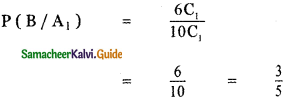Probability of selecting Urn – II
P(A2) = $$\frac{1}{2}$$
Conditional Probability of B, given A2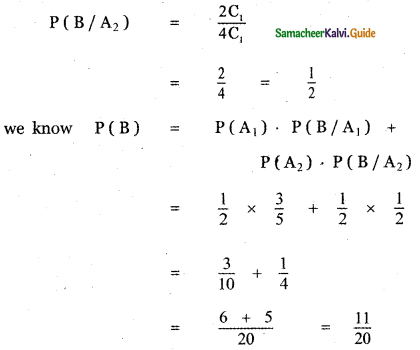(ii) The conditional Probability of A1 given B is P(A1/B)
By Bayes’ theorem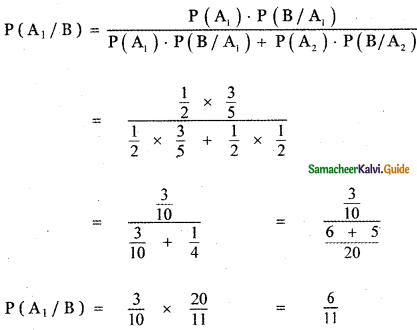Question 3.
A firm manufactures PVC pipes in the three plants viz, X, Y, and Z. The daily production volumes from the three firms X, Y, and Z are respectively 2000 units, 3000 units, and 5000 units. It is known from past experience that 3 % of the output from plant X, 4 % from plant Y, and 2 % from plant Z are defective. A pipe is selected at random from a day’s total production
(i) find the probability that the selected pipe is a defective one?
(ii) if the selected pipe is defective, then what is the probability that it was produced by plant Y?
Let A1 be the daily volume of production by plant X, A2 be the daily volume of production by plant Y, A3 be the daily volume of production by plant Z.
Let B be the defective output we have to find P (B).

(i) Find the probability that the selected pipe is a defective one:
Clearly, A1, A2, and A3 are mutually exclusive and exhaustive events.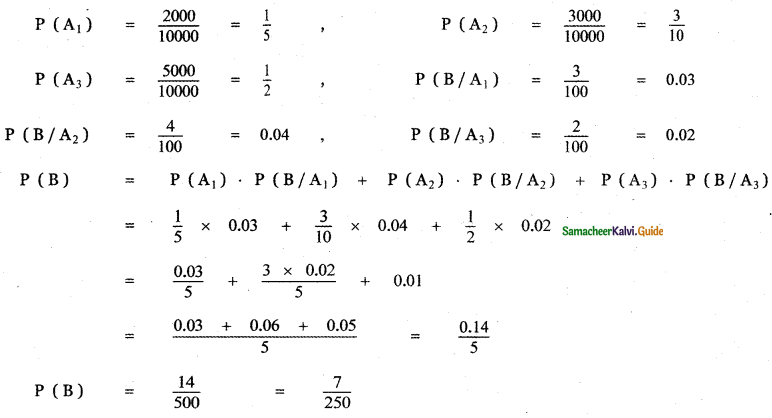Probability that the selected pipe is a defective one = $$\frac{7}{250}$$(ii) If the selected pipe is defective, then what is the probability that it was produced by plant Y?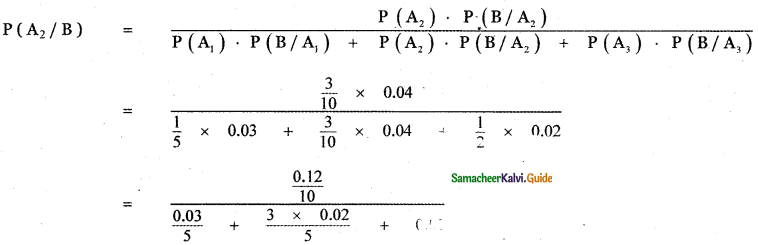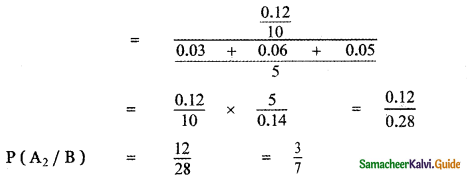Probability that the defective pipe produced by plant Y = $$\frac{3}{7}$$Question 4.
The chances of A, B, and C becoming manager of a certain company are 5 : 3 : 2. The probabilities that the office canteen will be improved if A, B, and C become managers are 0.4, 0.5 and 0.3 respectively. If the office canteen has been improved, what is the probability that B was appointed as the manager?
Let A1, A2, and A3 be the events of A, B, and C becoming managers of the company respectively.
Let B be the event that the office canteen will be improved.
We have to find the conditional probability P (A2/B).
Since A1, A2 and A3 are mutually exclusive and exhaustive events, applying Bayes theorem.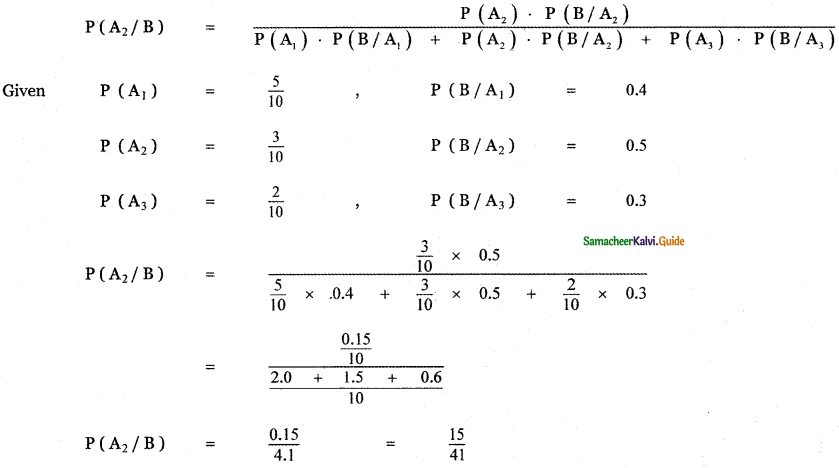If the office canteen is improved than the probability of that B was appointed as the manager is $$\frac{15}{41}$$Question 5.
An advertising executive is studying television viewing habits of married men and women during prime time hours. Based on the past viewing records he has determined that during prime time wives are watching television 60 % of the time. It has also been determined that when the wife is watching television, 40 % of the time the husband is also watching. When the wife is not watching the television, 30 % of the time the husband is watching the television. Find the probability that
(i) the husband is watching the television during the prime time of television
(ii) if the husband is watching the television, the wife is also watching the television.